# 毛发绘制（Fur Rendering）

## Rendering with shells

shell rendering的主要思路可由下图表示。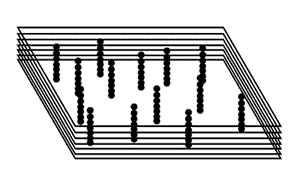• 毛发所处位置的信息。当绘制每个切片时，我们需要知道哪些像素是可见的（即属于直立的毛发的），哪些像素是透明的。我们将这个信息存储在一张纹理中。这个纹理中不透明的像素表示一个直立的毛发。当创建这个纹理时，我们会在其上随机放置不透明的像素，像素的数量是由一个表示毛发密度的值决定的。
• 切片的位置。如果只是制作一个简单的2D水平面上的毛发，切片的位置只是简单的沿着Up轴方向。但是，我们想在任意模型表面生成毛发，因此最好的方法就是让每个切片基于多边形的法线设置。事实上，要创建切片，我们只需绘制每个多边形几次，在每次绘制中，基于多边形的法线在一个很小的距离上显示多边形。
• 每根毛发的颜色。一开始，我们只是简单地从密度贴图读取颜色，但是到后面，我们将会从一个独立的贴图中读取颜色，这样可以实现一个漂亮的毛发效果。

## 生成毛发贴图（fur map）

///
/// 用于生成毛发绘制的纹理
///
/// 最终的毛发纹理
/// 位于[0..1]区间的毛发密度
private void FillFurTexture(Texture2D furTexture, float density)
{
// 读取纹理的长和宽
int width = furTexture.Width;
int height = furTexture.Height;

int totalPixels = width * height;

//保存像素的数组
Color[] colors;
colors = new Color[totalPixels];

// 随机数生成器
Random rand = new Random();

// 将像素的颜色初始化为transparent black
for (int i = 0; i < totalPixels; i++)
colors[i] = Color.TransparentBlack;

// 计算不透明像素的数量，即直立毛发的数量
int nrStrands = (int)(density * totalPixels);

// 使用不透明像素填充纹理
for (int i = 0; i < nrStrands; i++)
{
int x, y;
// 获取随机位置
x = rand.Next(height);
y = rand.Next(width);
// 施加颜色(alpha值为255)
colors[x * width + y] = Color.Gold;
}

// 将像素施加到纹理上。
furTexture.SetData(colors);
}

## 生成几何体

VertexPositionNormalTexture[] vertices;
private void GenerateGeometry()
{
vertices = new VertexPositionNormalTexture;
vertices = new VertexPositionNormalTexture(new Vector3(-10,0,0),  -Vector3.UnitZ,  new Vector2(0,0));
vertices = new VertexPositionNormalTexture(new Vector3(10,20,0),  -Vector3.UnitZ,  new Vector2(1,1));
vertices = new VertexPositionNormalTexture(new Vector3(-10, 20, 0), -Vector3.UnitZ, new Vector2(0, 1));
vertices = vertices;
vertices = new VertexPositionNormalTexture(new Vector3(10, 0, 0),-Vector3.UnitZ, new Vector2(1, 0));
vertices = vertices;
}

## 绘制shells

float4x4 World;
float4x4 View;
float4x4 Projection;

float CurrentLayer; //位于0和1之间的值
float MaxHairLength; //最大毛发长度

texture FurTexture;

sampler FurSampler = sampler_state
{
Texture = (FurTexture);
MinFilter = Point;
MagFilter = Point;
MipFilter = Point;
};

struct VertexShaderInput
{
float3 Position : POSITION0;
float3 Normal : NORMAL0;
float2 TexCoord : TEXCOORD0;
};

{
float4 Position : POSITION0;
float2 TexCoord : TEXCOORD0;
}; 

float3 pos = input.Position + input.Normal * MaxHairLength * CurrentLayer;

VertexShaderOutput FurVertexShader(VertexShaderInput input)
{

float3 pos;
pos = input.Position + input.Normal * MaxHairLength * CurrentLayer;

float4 worldPosition = mul(float4(pos,1), World);
float4 viewPosition = mul(worldPosition, View);
output.Position = mul(viewPosition, Projection);
output.TexCoord = input.TexCoord;

return output;
}

float4 FurPixelShader(VertexShaderOutput input) : COLOR0
{
return tex2D(FurSampler, input.TexCoord);
}

technique Fur
{
pass Pass1
{
AlphaBlendEnable = true;
SrcBlend = SRCALPHA;
DestBlend = INVSRCALPHA;
CullMode = None;
}
}

## 整合在一起：绘制几何体

private void DrawGeometry()
{
using (VertexDeclaration vdecl = new VertexDeclaration(GraphicsDevice,  VertexPositionNormalTexture.VertexElements))
{
GraphicsDevice.VertexDeclaration = vdecl;
GraphicsDevice.DrawUserPrimitives<VertexPositionNormalTexture>(PrimitiveType.TriangleList, vertices, 0, 2);
}
}

[...]
using XNASimpleCamera; //namespace of Camera.cs
[...]

public class Game1 : Microsoft.Xna.Framework.Game
{
[...]

// 相机
Camera camera;

// 包含毛发数据的纹理
Texture2D furTexture;

Effect furEffect;

// 毛发层数量
int nrOfLayers = 40;

// 毛发的最大长度
float maxHairLength = 2.0f;

// 毛发密度
float density = 0.2f;

protected override void Initialize()
{
// 初始化相机
camera = new Camera(this);
base.Initialize();
}

{
[...]
// 生成几何体
GenerateGeometry();

// 加载effect
(“FurEffect”);

// 创建纹理
furTexture = new Texture2D(GraphicsDevice,  256, 256, 1,  TextureUsage.None,  SurfaceFormat.Color);

//填充纹理
FillFurTexture(furTexture, density);
}
}

protected override void Draw(GameTime gameTime)
{
graphics.GraphicsDevice.Clear(Color.CornflowerBlue);

furEffect.Parameters[“World”].SetValue(Matrix.CreateTranslation(0,-10,0));
furEffect.Parameters[“View”].SetValue(camera.View);
furEffect.Parameters[“Projection”].SetValue(camera.Projection);
furEffect.Parameters[“MaxHairLength”].SetValue(maxHairLength);
furEffect.Parameters[“FurTexture”].SetValue(furTexture);

furEffect.Begin();
for (int i = 0; i < nrOfLayers; i++)
{
furEffect.Parameters[“CurrentLayer”].SetValue((float)i/nrOfLayers);
furEffect.CommitChanges();

furEffect.CurrentTechnique.Passes.Begin();
DrawGeometry();
furEffect.CurrentTechnique.Passes.End();
}
furEffect.End();
}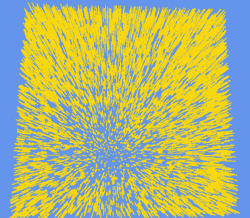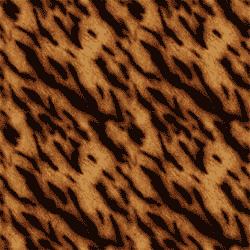Texture2D furColorTexture;
{
[...]
}

texture Texture;
sampler FurColorSampler = sampler_state
{
Texture = (Texture);
MinFilter = Linear;
MagFilter = Linear;
MipFilter = Linear;
};
[...]

{
float4 furData = tex2D(FurSampler, input.TexCoord);
float4 furColor = tex2D(FurColorSampler, input.TexCoord);

// 最下面的层是不透明的，否则透明值取自furData的alpha通道
furColor.a = (CurrentLayer == 0) ? 1 : furData.a;
return furColor;
}

furEffect.Parameters[“Texture”].SetValue(furColorTexture);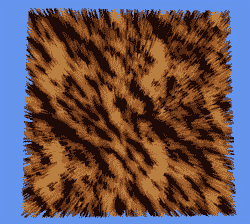## 改进

### 改进1:毛发间的遮挡效果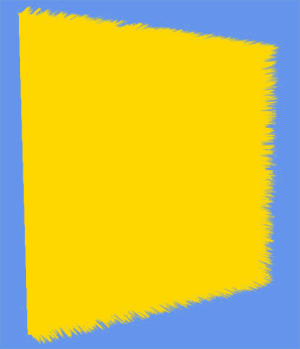// 基于层深度选择阴影程度。
// 我们对两个值进行插值避免底部的层漆黑一片
furColor *= shadow;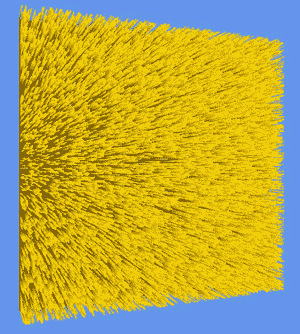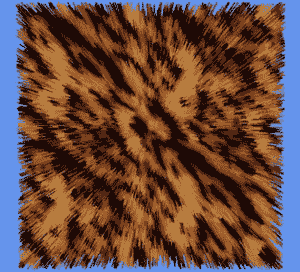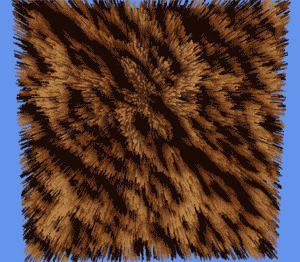### 改进2：高度变化

private void FillFurTexture(Texture2D furTexture, float density)
{
[...]

// 计算不透明像素的数量，即毛发的数量
int nrStrands = (int)(density * totalPixels);

// 计算达到每层的毛发数量
int strandsPerLayer = nrStrands / nrOfLayers;

// 使用不透明数据填充纹理
for (int i = 0; i < nrStrands; i++)
{
int x, y;
// 随机选择纹理上的位置
x = rand.Next(height);
y = rand.Next(width);

// 计算最大层
int max_layer = i / strandsPerLayer;
// 映射到[0..1]区间
float max_layer_n = (float)max_layer / (float)nrOfLayers;

// 填充颜色(alpha值为255，即不透明)
// max_layer_n需要乘以255映射到[0..255]区间
colors[x * width + y] = new Color((byte)(max_layer_n * 255), 0, 0, 255);
}
// 设置纹理上的像素数据
furTexture.SetData(colors);
}

float furVisibility =(CurrentLayer> furData.r) ? 0 : furData.a;
furColor.a = (CurrentLayer == 0) ? 1 : furVisibility;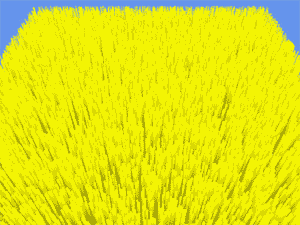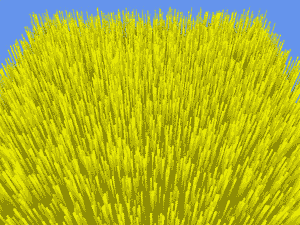max_layer_n = (float)Math.Sin(max_layer_n);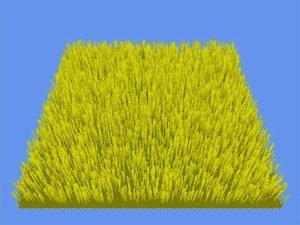max_layer_n = (float)Math.Pow(max_layer_n,5);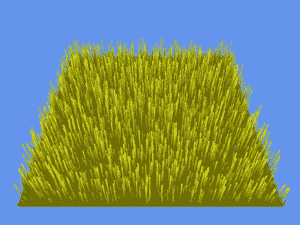max_layer_n = (float)Math.Sqrt(max_layer_n);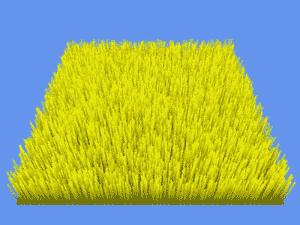if ((max_layer_n> 0.05f)&& (max_layer_n<0.95f))
max_layer_n = 0.5f;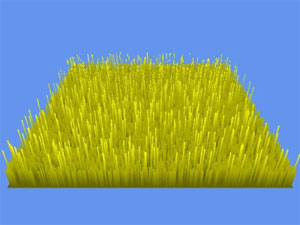max_layer_n = 0.2f + 0.8f * ((float)Math.Sin((double)x / height * 20) / 2.0f + 0.5f);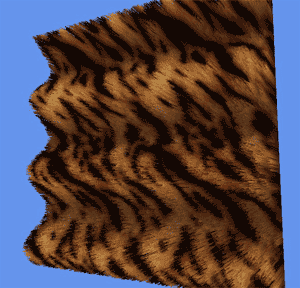Vector2 dist = new Vector2((float)x / height - 0.5f, (float)y / width - 0.5f);
max_layer_n = 0.4f + 0.6f *( (float)Math.Cos(dist.Length() * 50) / 2.0f + 0.5f);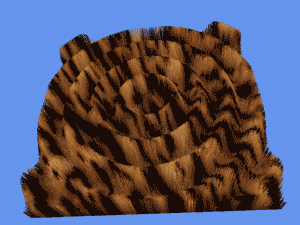### 改进3:变形

// 运动向量
Vector3 gravity = new Vector3(0, -1.0f, 0);
Vector3 forceDirection = Vector3.Zero;

// 毛发的最终位移
Vector3 displacement; 

float3 Displacement;

VertexShaderOutput FurVertexShader(VertexShaderInput input)
{
float3 pos;
pos = input.Position + input.Normal * MaxHairLength * CurrentLayer;
float4 worldPosition = mul(float4(pos,1), World);

// 给偏移施加非线性变化，让它更像毛发
float displacementFactor = pow(CurrentLayer, 3);
// 施加偏移
worldPosition.xyz +=Displacement*displacementFactor ;

[...]
return output;
}

displacement = gravity + forceDirection;
furEffect.Parameters[“Displacement”].SetValue(displacement);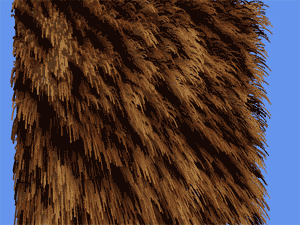forceDirection.X = (float)Math.Sin(gameTime.TotalGameTime.TotalSeconds) * 0.5f;

## 给模型添加毛发

//dino模型
Model dino;
{
}

private void DrawModelGeometry(Model model, Matrix[] bones, Effect effect)
{
foreach (ModelMesh mesh in model.Meshes)
{
// 设置World矩阵
effect.Parameters[“World”].SetValue(bones[mesh.ParentBone.Index]);
foreach (ModelMeshPart meshpart in mesh.MeshParts)
{
effect.Parameters[“Texture”].SetValue(((BasicEffect)meshpart.Effect).Texture);

// 设置纹理
effect.CommitChanges(); //commit changes
graphics.GraphicsDevice.VertexDeclaration = meshpart.VertexDeclaration;
graphics.GraphicsDevice.Vertices.SetSource(mesh.VertexBuffer, meshpart.StreamOffset, meshpart.VertexStride);
graphics.GraphicsDevice.Indices = mesh.IndexBuffer;
// 绘制模型
graphics.GraphicsDevice.DrawIndexedPrimitives(PrimitiveType.TriangleList, meshpart.BaseVertex, 0, meshpart.NumVertices, meshpart.StartIndex, meshpart.PrimitiveCount);
}
}
}

private void DrawFurModel(Model model)
{
Matrix[] bones = new Matrix[model.Bones.Count];
model.CopyAbsoluteBoneTransformsTo(bones);

furEffect.Parameters[“Displacement”].SetValue(displacement);
furEffect.Parameters[“MaxHairLength”].SetValue(maxHairLength);
furEffect.Parameters[“FurTexture”].SetValue(furTexture);

furEffect.Parameters[“View”].SetValue(camera.View);
furEffect.Parameters[“Projection”].SetValue(camera.Projection);
furEffect.Parameters[“MaxHairLength”].SetValue(maxHairLength);
furEffect.Parameters[“FurTexture”].SetValue(furTexture);

furEffect.Begin();
for (int i = 0; i < nrOfLayers; i++)
{
furEffect.Parameters[“CurrentLayer”].SetValue((float)i / nrOfLayers);
furEffect.CommitChanges();
furEffect.CurrentTechnique.Passes.Begin();
//绘制当前层的几何体
DrawModelGeometry(model, bones, furEffect);
furEffect.CurrentTechnique.Passes.End();
}
furEffect.End();
}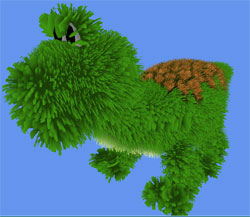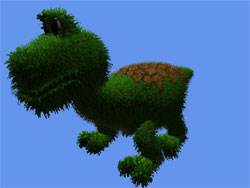2006 - 2023，推荐分辨率1024*768以上，推荐浏览器Chrome、Edge等现代浏览器，截止2021年12月5日的访问次数：1872万9823 站长邮箱